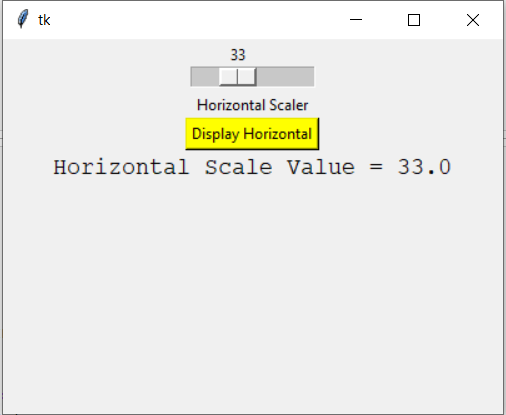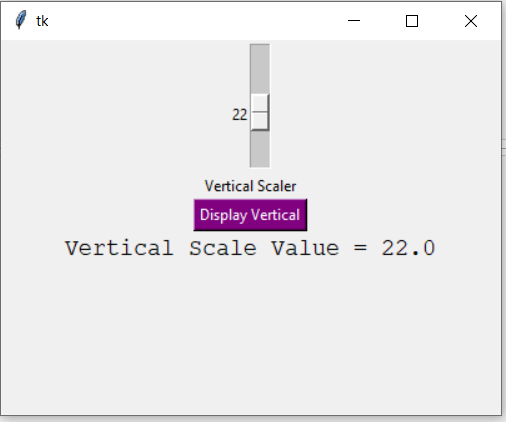# Python Tkinter – Scale Widget

• Last Updated : 26 Mar, 2020

Tkinter is a GUI toolkit used in python to make user-friendly GUIs.Tkinter is the most commonly used and the most basic GUI framework available in python. Tkinter uses an object-oriented approach to make GUIs.

Attention geek! Strengthen your foundations with the Python Programming Foundation Course and learn the basics.

To begin with, your interview preparations Enhance your Data Structures concepts with the Python DS Course. And to begin with your Machine Learning Journey, join the Machine Learning - Basic Level Course

## Scale widget

The Scale widget is used whenever we want to select a specific value from a range of values. It provides a sliding bar through which we can select the values by sliding from left to right or top to bottom depending upon the orientation of our sliding bar.

Syntax:

```S = Scale(root, bg, fg, bd, command, orient, from_, to, ..)
```

Optional parameters

• root – root window.
• bg – background colour
• fg – foreground colour
• bd – border
• orient – orientation(vertical or horizontal)
• from_ – starting value
• to – ending value
• troughcolor – set colour for trough.
• state – decides if the widget will be responsive or unresponsive.
• sliderlength – decides the length of the slider.
• label – to display label in the widget.
• highlightbackground – the colour of the focus when widget is not focused.
• cursor – The cursor on the widget ehich could be arrow, circle, dot etc.

Methods

• set(value) – set the value for scale.
• get() – get the value of scale.

Example 1: Creating a horizontal bar

 `# Python program to demonstrate ` `# scale widget ` ` `  `from` `tkinter ``import` `*`  ` `  ` `  `root ``=` `Tk()   ` `root.geometry(``"400x300"``)  ` ` `  `v1 ``=` `DoubleVar() ` ` `  `def` `show1():   ` `     `  `    ``sel ``=` `"Horizontal Scale Value = "` `+` `str``(v1.get()) ` `    ``l1.config(text ``=` `sel, font ``=``(``"Courier"``, ``14``))   ` ` `  ` `  `s1 ``=` `Scale( root, variable ``=` `v1,  ` `           ``from_ ``=` `1``, to ``=` `100``,  ` `           ``orient ``=` `HORIZONTAL)    ` ` `  `l3 ``=` `Label(root, text ``=` `"Horizontal Scaler"``) ` ` `  `b1 ``=` `Button(root, text ``=``"Display Horizontal"``,  ` `            ``command ``=` `show1,  ` `            ``bg ``=` `"yellow"``)   ` ` `  `l1 ``=` `Label(root) ` ` `  ` `  `s1.pack(anchor ``=` `CENTER)  ` `l3.pack() ` `b1.pack(anchor ``=` `CENTER) ` `l1.pack()  ` ` `  `root.mainloop() `

Output:Example 2: Creating a vertical slider

 `from` `tkinter ``import` `*` ` `  `root ``=` `Tk()   ` `root.geometry(``"400x300"``)  ` `v2 ``=` `DoubleVar() ` ` `  `def` `show2(): ` `     `  `    ``sel ``=` `"Vertical Scale Value = "` `+` `str``(v2.get())  ` `    ``l2.config(text ``=` `sel, font ``=``(``"Courier"``, ``14``)) ` ` `  `s2 ``=` `Scale( root, variable ``=` `v2, ` `           ``from_ ``=` `50``, to ``=` `1``, ` `           ``orient ``=` `VERTICAL)  ` ` `  `l4 ``=` `Label(root, text ``=` `"Vertical Scaler"``) ` ` `  `b2 ``=` `Button(root, text ``=``"Display Vertical"``, ` `            ``command ``=` `show2, ` `            ``bg ``=` `"purple"``,  ` `            ``fg ``=` `"white"``) ` ` `  `l2 ``=` `Label(root) ` ` `  `s2.pack(anchor ``=` `CENTER)  ` `l4.pack() ` `b2.pack() ` `l2.pack() ` ` `  `root.mainloop() `

Output:My Personal Notes arrow_drop_up
Recommended Articles
Page :